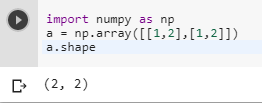2 views
in Python

I'm currently trying to learn Numpy and Python. Given the following array:

import numpy as np

a = np.array([[1,2],[1,2]])

Is there a function that returns the dimensions of an (e.g.a is a 2 by 2 array)?

size() returns 4 and that doesn't help very much.

by (106k points)

To get Numpy array’s dimensions you can use shape() function. The output of the dimension will be in the form of the tuple:

An example that shows how to do it:-

import numpy as np

a = np.array([[1,2],[1,2]])

a.shape